# 11. Iteration¶

Computers are often used to automate repetitive tasks. Repeating identical or similar tasks without making errors is something that computers do well and people do poorly.

Repeated execution of a set of statements is called iteration. Because iteration is so common, C# provides several language features to make it easier. We’ve already seen the `while` statement. But in this chapter we’ve going to look at the `for` and `foreach` statements again — another way to have your program do iteration, useful in slightly different circumstances.

Before we do that, let’s review a few ideas...

## 11.1. Assignment¶

As we have mentioned previously, as the program runs over time, it is legal to make more than one assignment to the same variable. A new assignment gives an existing variable a new value (and it loses its old value). Of course, the variable must already have been defined before we can assign to it. C# also allows us to give a newly defined variable a value right at the time we define it — we call this an initializer. Using initializers is considered good practice.

 ```1 2 3 4``` ```int airtime_remaining = 1500; Console.WriteLine(airtime_remaining); airtime_remaining = 700; Console.WriteLine(airtime_remaining); ```

The output of this program on the Console is:

```1500
700
```

because the first time `airtime_remaining` is printed, its value is 1500, and the second time, its value is 700.

It is especially important to distinguish between an assignment statement and a Boolean expression that tests for equality. Because C# uses the equal token (`=`) for assignment, it is tempting to interpret a statement like `a = b` as a Boolean test. Unlike mathematics, it is not! Remember that the C# token for the equality operator is `==`.

Note too that an equality test is symmetric, but assignment is not. For example, if `a == 7` then `7 == a`. But in C#, the assignment `a = 7` is legal but `7 = a` is not.

In C#, an assignment statement can make two variables equal, but because further assignments can change either of them, they don’t have to stay that way:

 ```1 2 3``` ```int a = 5; int b = a; // After executing this line, a and b are now equal a = 3; // After executing this line, a and b are no longer equal ```

The third line changes the value of `a` but does not change the value of `b`, so they are no longer equal. (In some programming languages, a different symbol is used for assignment, such as `<-` or `:=`, to avoid confusion. Some people also think that variable was an unfortunate word to choose, and instead we should have called them assignables. C# chooses to follow common terminology and token usage, also found in languages like C, C++, Java, and Python, so we use the tokens `=` for assignment, `==` for equality, and we talk of variables.

## 11.2. Updating variables¶

When an assignment statement is executed, the right-hand side expression (i.e. the expression that comes after the assignment token) is evaluated first. This produces a value. Then the assignment is made, so that the variable on the left-hand side now holds the new value.

One of the most common forms of assignment is an update, where the new value of the variable depends on its old value.

 ```1 2 3 4 5 6``` ```//Deduct 40 cents from my airtime balance. airtime_remaining = airtime_remaining - 0.40; int n = 5; // Change n, based on it's current value. n = (n % 2 == 0) ? n / 2 : 3 * n + 1; ```

When reading an assignment statement, develop the habit of evaluating the right hand side first, because this is how computer languages do it. So line 6 means first get the current value of n and evaluate the conditional expression to test if it is even. Since it is not even, multiply it by three and then add one. (This results in the value 16 in this case.) Then assign that 16 to the variable n.

If we try to get the value of a variable that has never been assigned to, (and if the compiler is not clever enough to understand the situation) we’ll get its default initialization value. This is the value that C# gives the variable if we do not provide an initializing expression. For numbers the initial value is zero, for strings it is the value `null`.

Some programming languages don’t provide initializing values automatically, and our variables could start off containing random nonsense. For this reason it is a good habit to make sure that we explicitly give every variable an initial value.

The C# compiler can sometimes spot that we have never initialized a variable, and it will give an error if we try to use it. But it doesn’t always catch this. And if we inspect the variable values in memory using the debugger, we’ll see that they have been initialized to their default initialization values.

The automatic initialization feature will become more useful when we cover arrays in the subsequent chapters. We can define an array of a thousand integers, and we can safely assume that they will all have their initial value of zero.

## 11.3. Abbreviated assignment¶

Adding something to a variable is so common that C# provides an abbreviated syntax for it: `count += 4;` is an abbreviation for `count = count + 4;` We pronounce the operator as “plus-equals”.

```runs_scored += 4;
```

There are similar abbreviations for `-=`, `*=`, `/=` and `%=`.

What is the final value of `n` after executing this sequence of statements?

```int n = 123;
n -= 10;
n *= 2;
n /= 3;
n %= 7;
```

Incrementing (adding one to) a variable (we say we bump the variable) or decrementing (subtracting one from) a variable is also so common that C# provides more special shorthand:

```n++;      // Adds one to n
k--;      // Subtracts one from k
```

C is an older language that uses this shorthand. C evolved into a new language called C++ (a clever name, suggesting that C++ is “one better than C”). C# is a great name for a language too. It says “We’re also related to C, but we’re sharper!”. (In music, C# (C sharp) is one semitone above C.)

## 11.4. The `foreach` loop revisited¶

Recall that the `foreach` loop processes each item in an array. Each item in turn is (re-)assigned to the loop variable, and the body of the loop is executed. Running through all the items in an array is called traversing the items.

Let us write a method to sum up all the elements in an array of numbers. We should do this by hand first, and try to isolate exactly what steps we take. We’ll find we need to keep track of some “running total” of the sum so far, either on a piece of paper, in our head, or in our calculator. Remembering things from one step to the next is precisely why we have variables in a program, and why we have a memory in a calculator: so our program will need a variable to remember the “running total”. It should be initialized to the value zero. As we traverse the items in the array, we’ll update the running total by adding the current item to the running total.

 ``` 1 2 3 4 5 6 7 8 9 10 11 12 13 14 15 16 17 18``` ```private int mySum(int[] xs) { // Sum all the numbers in the array xs, and return the total. int running_total = 0; foreach (int x in xs) { running_total += x; } return running_total; } // Add tests like these to your test suite ... int[] ws = {1, 2, 3, 4}; Tester.TestEq(mySum(ws), 10); int[] ys = {1, -2, 3}; Tester.TestEq(mySum(ys), 2); int[] zs = { }; // notice this case. foreach works fine with an empty array. Tester.TestEq(mySum(zs), 0); ```

## 11.5. The `for` loop¶

C# comes with extensive documentation for all its built-in methods, and its libraries. Different systems have different ways of accessing this help. In Visual Studio, you can put your mouse cursor above a keyword like `for` and hit the `F1` key to get help. Learn to read and make frequent use of the documentation — it takes a little getting used to. We’ll use Microsoft’s documentation here instead of writing our own: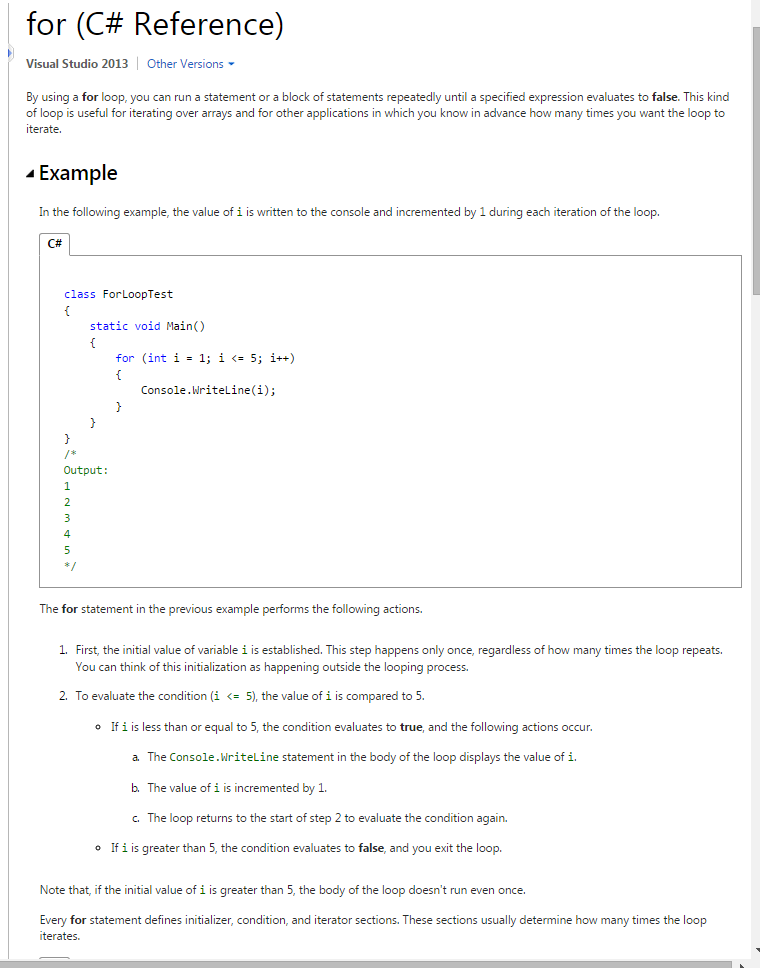## 11.6. The `while` statement¶

Let us now write a method to calculate the sum of all the numbers between 1 and N. There are at least two ways to do this: perhaps we know the formula for the answer, and can just plug N into the formula. Or, on the other hand, perhaps we’ll just solve it the long way: write a loop and total them all up one at a time. Let’s start with a method for doing this that demonstrates the use of the `while` statement:

 ``` 1 2 3 4 5 6 7 8 9 10 11 12 13 14 15``` ```private int sumTo(int n) { // Return the sum of 1+2+3 ... n int ss = 0; int v = 1; while (v <= n) { ss += v; v++; } return ss; } // For our test suite Tester.TestEq(sumTo(4), 10); Tester.TestEq(sumTo(1000), 500500); ```

We can almost read the `while` statement as if it were English. It means, while `v` is less than or equal to `n`, continue executing the body of the loop. Within the body, each time, increment `v`. When `v` passes `n`, return our accumulated sum.

More formally, here is precise flow of execution for a `while` statement:

• Evaluate the condition at line 5, yielding a value which is either `false` or `true`.
• If the value is `false`, exit the `while` statement and continue execution at the next statement (line 10 in this case).
• If the value is `true`, execute each of the statements in the body (lines 7 and 8) and then go back to the `while` statement at line 5.

The body of the loop consists of all of the statements within the braces after the `while` keyword.

Notice that if the loop condition is `false` the first time we get loop, the statements in the body of the loop are never executed. (What test case could you write if you didn’t want the body of the loop to execute at all?)

Usually the body of the loop will change the value of one or more variables so that eventually the controlling condition becomes false and the loop terminates. Otherwise the loop will repeat forever. This is called an infinite loop.

In the case here, we can easily reason that the loop terminates because we know that the value of `n` is finite, and we can see that the value of `v` increments each time through the loop, so eventually it will have to exceed `n`. In other cases, it is not so easy, sometimes even impossible, to prove, that a loop will or will not terminate.

What we notice in the previous example is that the `while` loop is more work for us — the programmers — than the equivalent `for` loop. When using a `while` loop one has to manage the loop variable yourself: define it, give it an initial value, test for completion, and then make sure you change something in the body so that the loop terminates. By comparison, here is a method that uses `for` instead:

 ```1 2 3 4 5 6 7 8 9``` ```private int sumTo(int n) { // Return the sum of 1+2+3 ... n int ss = 0; for (int v = 1; v <= n; v++) { ss += v; } return ss; } ```

There is also a simple formula for doing this really fast, without the need for N iterations. Check out http://en.wikipedia.org/wiki/Summation and make sure you can also write a C# method to compute `sumTo` without using any looping at all!

So why have a `while` loop if a `for` loop seems easier? This next example shows a case where we need the extra power that we get from the `while` loop.

## 11.7. The Collatz 3n + 1 sequence (AKA the Hailstone sequence)¶

Let’s look at a simple sequence that has fascinated and foxed mathematicians since 1937. They still cannot answer even quite simple questions about this.

The “computational rule” for creating the sequence is to start from some given `n`, and to generate the next term of the sequence from `n`, either by halving `n`, (whenever `n` is even), or else by multiplying it by three and adding 1. The sequence terminates when `n` reaches 1. Start by doing some sequences by hand or by using your calculator.

This C# method captures that algorithm:

 ``` 1 2 3 4 5 6 7 8 9 10 11 12 13 14 15 16 17 18``` ```private void collatz(int n) { // Print the 3n+1 sequence starting from n, // terminating when it reaches 1. while (n != 1) { Console.Write("{0}, ", n); if (n % 2 == 0) // n is even { n /= 2; } else // n is odd { n = n * 3 + 1; } } Console.WriteLine("{0}. Yes, it got to 1!", n); } ```

Notice first that lines 7 and 17 perform output, this time to the console. If you’re working in Visual Studio, use View | Output to see your answers. If we pass the value 24 to the method, your Console output should look like this: (Visual Studio outputs a lot of other stuff into the same Console window, so you may need to hunt for your answers in the middle of other noise!)

```24, 12, 6, 3, 10, 5, 16, 8, 4, 2, 1. Yes, it got to 1!
```

Of course you might prefer to send your output to your GUI instead of having to search for the Console window, and search for the text within that window. You could replace lines 7 and 17 above with these two lines:

 ```1 2 3``` ``` txtResult.AppendText(n.ToString() + ", "); ... txtResult.AppendText(n.ToString() + ". Yes, it got to 1!\n"); ```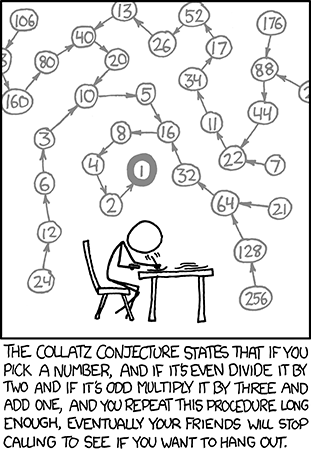The condition for continuing with this loop is `n != 1`, so the loop will continue running until it reaches its termination condition, (i.e. `n == 1`).

Each time through the loop, the program outputs the value of `n` and then checks whether it is even or odd. If it is even, the value of `n` is divided by 2 using integer division. If it is odd, the value is replaced by `n * 3 + 1`.

Since `n` sometimes increases and sometimes decreases, there is no obvious proof that `n` will ever reach 1, or that the program terminates. For some particular values of `n`, we can prove termination. For example, if the starting value is a power of two, then the value of `n` will be even each time through the loop until it reaches 1. The previous example ends with such a sequence, starting with 16.

See if you can find a small starting number that needs more than a hundred steps before it terminates.

Particular values aside, the interesting question was first posed by a German mathematician called Lothar Collatz: the Collatz conjecture (also known as the 3n + 1 conjecture), is that this sequence terminates for all positive values of `n`. So far, no one has been able to prove it or disprove it! (A conjecture is a statement that might be true, but nobody knows for sure.)

Think carefully about what would be needed for a proof or disproof of the conjecture “All positive integers will eventually converge to 1 using the Collatz rules”. With fast computers we have been able to test every integer up to very large values, and so far, they have all eventually ended up at 1. But who knows? Perhaps there is some as-yet untested number which does not reduce to 1.

You’ll notice that if you don’t stop when you reach 1, the sequence gets into its own cyclic loop: 1, 4, 2, 1, 4, 2, 1, 4 ... So one possibility is that there might be other cycles that we just haven’t found yet.

Wikipedia has an informative article about the Collatz conjecture. The sequence also goes under other names (Hailstone sequence, Wonderous numbers, etc.), and you’ll find out just how many integers have already been tested by computer, and so far, every one has been found to converge to 1!

Choosing between `for` and `while`

Use a `for` loop if you know, before you start looping, the maximum number of times that you’ll need to execute the body. For example, if you’re traversing an array of elements, you know that the maximum number of loop iterations you can possibly need is “all the elements in the array”. Or if you need to print the 12 times table, we know right away how many times the loop will need to run.

So any problem like “iterate this weather model for 1000 cycles”, or “search this array of words”, “find all prime numbers up to 10000” suggests that a `for` loop is better.

By contrast, if you are required to repeat some computation until some condition is met, and you cannot calculate in advance when (or if) this will happen, (as was the case in this 3n + 1 problem), you’ll need a `while` loop.

We call the first case definite iteration — we know ahead of time some definite bounds for what is needed. The latter case is called indefinite iteration — we’re not sure how many iterations we’ll need — we cannot even establish an upper bound!

## 11.8. Counting¶

We often want to count something: perhaps the number of times we had to iterate a Collatz sequence number before we reached one. The pattern for counting has three parts:

• define and initialize a counter to 0 before your iteration or before the region of code that needs the counting operation,
• in the loop body or repeated code, increment the counter each time you want to count another item,
• when the loop terminates, your counter contains the count you want.

Sometimes you’ll be asked to only count items that satisfy some condition: how many students passed the test, or how many numbers are odd. So you’ll put the increment statement under a conditional, so that it only gets counted sometimes.

We illustrate by changing our Collatz program to also count and show how many elements of the sequence were strictly greater than 10. The new bits of the program are highlighted. We’ve also got rid of the verbose `if` statement and used the more compact conditional expression in its place at line 11.

 ``` 1 2 3 4 5 6 7 8 9 10 11 12 13 14 15``` ```private void collatz(int n) { // Print the 3n+1 sequence starting from n, // terminating when it reaches 1. int bigNumCount = 0 while (n != 1) { Console.Write("{0}, ", n); if (n > 10) bigNumCount++; n = (n % 2 == 0) ? n / 2 : n * 3 + 1; } Console.WriteLine("{0}. Yes, it got to 1!", n); Console.WriteLine("{0} numbers were bigger than 10.", bigNumCount); } ```

## 11.9. Tracing a program¶

To write effective computer programs, and to build a good conceptual model of program execution, a programmer needs to develop the ability to trace the execution of a computer program. Tracing involves becoming the computer and following the flow of execution through a sample program run, recording the state of all variables and any output the program generates after each instruction is executed.

To understand this process, let’s trace the call to `collatz(3)` from the previous section. At the start of the trace, we have a variable, `n` (the parameter), with an initial value of 3. Since 3 is not equal to 1, the `while` loop body is executed. 3 is printed. At line 10, we see that `n` is not bigger than `n`, so nothing happens. Now the conditional expression at line 11 is executed: first its condition `(3 % 2 == 0)` is evaluated. Since it evaluates to `false`, the value of `3 * 3 + 1` is evaluated and assigned to `n`.

To keep track of all this as you hand trace a program, make a column heading on a piece of paper for each variable, and another column for output. On each line, record the values of the variables at the end of each iteration of the loop: Our trace so far would look something like this:

```n       bigNumCount        output so far
--      -----------        -------------
3                 0        3,
10
```

Since `10 != 1` evaluates to `true`, the loop body is again executed, and 10 is printed. `(10 % 2 == 0)` is true, so the first part of the conditional expression is evaluated and `n` becomes 5. By the end of the trace we have:

```n       bigNumCount       output so far
--      -----------       -------------
3                 0       3,
10                0       3, 10,
5                 0       3, 10, 5,
16                1       3, 10, 5, 16,
8                 1       3, 10, 5, 16, 8,
4                 1       3, 10, 5, 16, 8, 4,
2                 1       3, 10, 5, 16, 8, 4, 2,
1                 1       3, 10, 5, 16, 8, 4, 2, 1. Yes, it got to 1!
1 numbers were bigger than 10.
```

Tracing can be a bit tedious and error prone (that’s why we get computers to do this stuff in the first place!), but it is an essential skill for a programmer to have. From this trace we can learn a lot about the way our code works. We can observe that as soon as `n` becomes a power of 2, for example, the program will require log2(n) executions of the loop body to complete. We can also see that the final 1 will not be printed as output within the body of the loop, which is why we put the special statement at line 13.

Tracing a program is, of course, related to single-stepping through your code and being able to inspect the variables. Using the computer to single-step is less error prone and more convenient. Also, as your programs get more complex, they might execute many millions of steps before they get to the code that you’re really interested in, so manual tracing becomes impossible. Being able to set a breakpoint where you need one is far more powerful. So we strongly encourage you to invest time in learning using to use your programming environment (Visual Studio, in these notes) to full effect.

## 11.10. Counting digits¶

The following method counts the number of decimal digits in a positive integer:

 ``` 1 2 3 4 5 6 7 8 9 10``` ```private int num_digits(int n) { int count = 0; while (n != 0) { count++; n /= 10; } return count; } ```

Calling `num_digits(710)` will return the value `3`. Trace the execution of this method call (perhaps using the single step method in Visual Studio, or on some paper) to convince yourself that it works.

If we wanted to only count digits that are either 0 or 5, adding an `if` statement before incrementing the counter will do the trick:

 ``` 1 2 3 4 5 6 7 8 9 10 11 12 13 14``` ```private int num_zero_and_five_digits(int n) { int count = 0; while (n > 0) { int digit = n % 10; if ((digit == 0) || (digit == 5)) { count++; } n /= 10; } return count; } ```

Notice a clever technique in line 6 here. By finding the remainder after division by 10 we have isolated the last digit of the current number.

Confirm that `Tester.TestEq(num_zero_and_five_digits(1055030250), 7);` passes. Notice, however, that `Tester.TestEq(num_digits(0), 1);` fails. Explain why. Do you think this is a bug in the code, or a bug in the specifications, or our expectations, or the tests?

## 11.11. Tables¶

One of the things loops are good for is generating tables. Before computers were readily available, people had to calculate logarithms, sines and cosines, etc. by hand. To make that easier, mathematics books often contained long tables to let the reader look up the answer rather than calculate it from scratch. Creating the tables was slow and boring, and they tended to have occasional errors.

When computers appeared on the scene, one of the initial reactions was, “This is great! We can use the computers to generate the tables, so there will be no errors.” That turned out to be true (mostly) but short sighted. Soon thereafter, computers and calculators were so pervasive that the tables became obsolete, and we found more interesting uses for computers — YouTube, cellphones, games, etc.

For some operations, computers still use tables of values to get an approximate answer, and then they perform computations to improve the approximation. In some cases, there have been errors in the underlying tables, most famously in the tables that one generation of the Intel Pentium processor chip used to perform floating-point division.

Although a log or power table is not as useful as it once was, it still makes a good example of iteration. The following program outputs a sequence of values in the left column and 2 raised to the power of that value in the right column:

 ```1 2 3 4 5 6 7 8``` ```private void button1_Click(object sender, EventArgs e) { for (int i=1; i <= 12; i++) { string txt = string.Format("{0,4} {1,8}\n", i, Math.Pow(2, i)); txtResult.AppendText(txt); } } ```

Line 3 uses a `for` loop to run the body of the loop 12 times, while varying `i` between 1 and 12 (both inclusive). Notice that we’ve now used the abbreviated assignment `i++` — this is the typical way you’re going to see the loop written in other code. Line 5 does some magic with string formatting. We’ll be covering more about this in the next chapter, but here is a quick preview: The string.Format method in this case has 3 arguments: the first is a template string with “place-holders” `{0}` and `{1}`. The value of `i` is converted to a string and takes the place of the first place-holder. Then `Math.Pow(2, i)` computes 3 to the power of i, and it too gets converted to a string and takes the place of the second place-holder. The resulting string is assigned to `txt`, and we then find some way to display or output the text. (Of course, if you prefer working with the console you could have said `Console.Write(txt)` instead.)

In the example here, each place-holder has an extra bit of information about how wide the field should be to hold the number. This lets us make the columns in table line up nicely.

We’ll see output like this: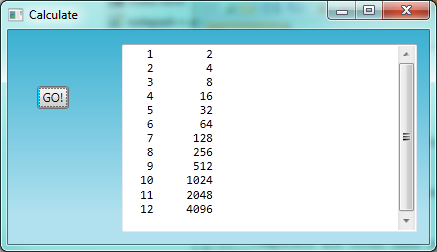Proportional vs Monospace fonts

If you try this, your output may initially look different. To lay out data in fixed columns, we need to make sure that every character that is output takes exactly the same number of pixels, or width. This kind of font is called a monospace font (or fixed-width font). Normal fonts, like this text you are reading, are called proportional fonts — thin characters like ‘i’ and ‘l’ take less width that wide characters like ‘w’ and ‘b’.

So in the example image above, we’ve set a property for `txtResult` to use the font Consolas. Other popular monospace fonts are the Courier family. See which you prefer. The Console output window in C# will always automatically use a monospace font, and program source code is usually shown in a monospace font.## 11.12. Two-dimensional tables¶

A two-dimensional table is a table where you can look up the value at the intersection of a row and a column. (A row in a table is laid out horizontally, on a single line. The columns go vertically down the page.) A multiplication table is a good example. Let’s say you want to create a multiplication table for the values from 1 to 6. (So it has 6 rows, and 6 columns.) Like this: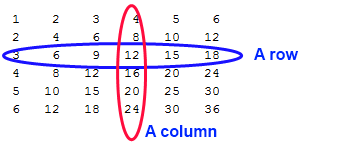A good way to start is to write a loop that generates one of the rows, say all the multiples of 2, all on one line:

 ```1 2 3 4 5``` ```for (int col=1; col <= 6; col++) { txtResult.AppendText(string.Format("{0,5}", 2 * col)); } txtResult.AppendText("\n"); ```

As the loop executes, the value of `col` changes from 1 to 6. When `col` gets larger than 6, the loop terminates, and we end off the line. Each value of `2 * col` uses a field width of 5 spaces.

Line 5 finishes the current line, and starts a new line. Once again, if you prefer working with the Console, you could have said `Console.Write(...)` on line 3, and `Console.WriteLine()` on line 5.

The output of the program is now the second row of the table we want to create:

```2    4    6    8   10   12
```

So far, so good. The next step is to encapsulate and generalize.

## 11.13. Encapsulation and generalization¶

Encapsulation is the process of wrapping a piece of code in a method, allowing you to take advantage of all the things methods are good for. You have already seen some examples of encapsulation, including `isDivisible` in a previous chapter.

Generalization means taking something specific, such as generating the multiples of 2, and making it more general, such as generating the multiples of any integer.

This method encapsulates the previous loop and generalizes it to create multiples of `n`:

 ```1 2 3 4 5 6 7 8``` ```private void generateOneRow(int n) { for (int col=1; col <= 6; col++) { txtResult.AppendText(string.Format("{0,5}", n * col)); } txtResult.AppendText("\n"); } ```

To encapsulate, all we had to do was add the signature, which defines the name of the method with it’s parameter. To generalize, all we had to do was replace the value 2 with the parameter `n`.

If we call this method with the argument 2, we get the same output as before. With the argument 3, the output is:

```3    6    9   12   15   18
```

With the argument 4, the output is:

```4    8   12   16   20   24
```

By now you can probably guess how to create our multiplication table — by calling `generateOneRow` repeatedly with different arguments. In fact, we can use another loop:

 ```1 2 3 4 5 6 7``` ```private void generateTable() { for (int row = 1; row <= 6; row++) { generateOneRow(row); } } ```

By calling this method we get the table shown at the beginning of this section. We called our loop variables `row` and `col`, but of course any legal identifier would have worked. But when you work with tables you’ll find programmers often choose these names (or if they’re a bit lazier, they might use `r` and `c`).

Exercise: can we generalize further?

The method `generateTable` can only generate a 6x6 table. What if we wanted a 10x10 table, or 12x12, or 50x50?

Add a new parameter to `generateTable` that controls how many rows to generate. When that works you’ll find each row still only has 6 columns. So generalize `generateOneRow` by adding another parameter to control how many columns to generate.

## 11.14. Local variables, revisited¶

Do the following experiment: change the variable called `row` and call it `i` instead. (Visual Studio understands when you rename a variable, and will offer to rename all other places where it is used. So it can help you make small cosmetic changes like this very easily.) Make sure your program still works as expected.

Now do the same for the `col` variable: change it to `i`. Does it still work?

You might be wondering how we can use the same variable, `i`, in both `generateTable` and `generateOneRow`. Doesn’t it cause problems when one of the methods changes the value of the variable `i`?

The answer is no, because the `i` in `generateTable` and the `i` in `generateTable` are not the same variable.

In C#, a number of statements grouped together (usually by curly braces) is called a block, or equivalently, a compound statement. Each block in a program can have its own local variable definitions, and these don’t clash with variables defined in other blocks.

So variables created inside a block (or a method) are local to the block (or method); you can’t access a local variable from outside its block where it is defined. That means you are free to have multiple variables with the same name as long as they are not in the same block. It also means that updating the variable `i` in `generateOneRow` has absolutely no effect on the variable `i` in `generateTable` — they are different variables, even though they have the same name! (You probably also know quite a few friends called John. Of course they’re different!)

In C# the `for` and `foreach` statements also let you define your loop control variable, and that is a local variable that only exists for the loop. So you’ll often find C# code that does something like this:

 ``` 1 2 3 4 5 6 7 8 9 10 11``` ```for (int i = 1; i <= 6; i++) { int p = 123 * i; // ... do stuff } for (int i = 1; i <= 15; i++) // Use i again, it is a different variable. { double p = 3.1415 * i; // Use p again, it is in a different block. // ... do more stuff } ```

The variables `i` in lines 1 and 7 are different variables. Similarly, the variable `p` at line 3 is not the same variable as the `p` at line 9.

It is common and perfectly legal to have different local variables with the same name. In particular, names like `i` and `j` are used frequently as loop variables. If you avoid using them in one method just because you used them somewhere else, you will probably make the program harder to read.

## 11.15. The `break` statement¶

The break statement is used to immediately leave the body of its loop. The next statement to be executed is the first one after the body:

 ``` 1 2 3 4 5 6 7 8 9 10``` ```int[] nums = {12, 16, 17, 24, 29, 30}; foreach (int n in nums) { if (n % 2 == 1) { break; // immediately exit the loop. } txtResult.AppendText(string.Format("{0} ", n)); } txtResult.AppendText("done\n"); ```

This will put the following text into `txtResult`:

```12 16 done
```

Notice that on the third iteration of the loop, `n` is assigned the value 17. So line 6 executes, and immediately the flow of control jumps to line 10: the 17 is not shown in the output. How would this be different if we moved the test at line 4 below the statement on line 8?

## 11.16. The `continue` statement¶

This is a control flow statement that causes the program to immediately skip the processing of the rest of the body of the loop, for the current iteration. But the loop still carries on running for its remaining iterations:

 ``` 1 2 3 4 5 6 7 8 9 10``` ```int[] nums = {12, 16, 17, 24, 29, 30}; foreach (int n in nums) { if (n % 2 == 1) { continue; // skip the rest of the loop for odd numbers. } txtResult.AppendText(string.Format("{0} ",n)); } txtResult.AppendText("done\n"); ```

This generates the output

```12 16 24 30 done
```

The pre-test loop — standard loop behaviour

`for`, `foreach` and `while` loops do their tests at the start, before executing any part of the body. They’re called pre-test loops, because the test happens before (pre) the body. `break`, `return` and `continue` are our tools for adapting this standard behaviour.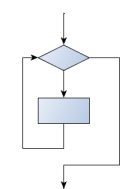## 11.17. More generalization¶

As another example of generalization, imagine you wanted a program that would print a multiplication table of any size, not just the six-by-six table. You could add a parameter to `generateMultiplicationTable`:

 ```1 2 3 4 5 6 7``` ```private void generateMultiplicationTable(int sz) { for (int i = 1; i <= sz; i++) { generateMultiples(i); } } ```

We replaced the value 6 with the expression `sz`. If we call `generateMultiplicationTable` with the argument 3, it displays:

```1    2    3    4    5    6
2    4    6    8   10   12
3    6    9   12   15   18
```

This is fine, except that we probably want the table to be square — with the same number of rows and columns. To do that, we add another parameter to `generateOneRow` to specify how many columns the table should have.

We also call this parameter `sz`, demonstrating that different methods can have parameters with the same name (just like local variables). Here’s the modified section of code.

 ``` 1 2 3 4 5 6 7 8 9 10 11 12 13 14 15 16 17 18 19 20 21 22``` ```private void generateMultiples(int n, int sz) { for (int i=1; i <= sz; i++) { txtResult.AppendText(string.Format("{0,5}",i*n)); } txtResult.AppendText("\n"); } private void generateMultiplicationTable(int sz) { for (int i = 1; i <= sz; i++) { generateMultiples(i, sz); } } private void button1_Click(object sender, RoutedEventArgs e) { int tsz = int.Parse(textBox1.Text); generateMultiplicationTable(tsz); } ```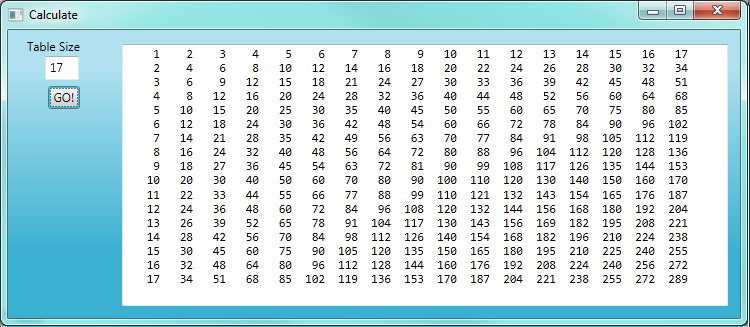We notice that, because `a*b == b*a`, the non-diagonal entries in the table appear twice. (Our mathematicians would call this a symmetric matrix.) We can highlight this important insight by only generating half the table. To do that, we’d only have to change one line of code — line 14:

 `1` ```generateMultiples(i, i); ```

and now, for a 7 times table, we’ll get: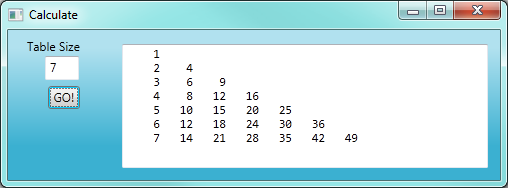## 11.18. Nested loops¶

C# has a very general approach to composibility: if it is legal to write a statement at a given point, then it is legal to write any kind of statement, or even a whole block of statements. What this means is that it is legal to put an `if` statement inside a `for` loop, or a `for` loop inside another `for` loop. When we put a loop inside another loop we say the loops are nested. Nested loops are often required to process two-dimensional data — like our table that we’ve just created, or some image processing that needs to look at all the pixels in an image.

We generated our multiplication table using two separate methods: that mental decomposition helped keep things simple at each step. But separate methods also introduce some overhead - we had to pass arguments between them, etc.

Here we generate the same triangular multiplication table again, but this time we only use a single method with a nested loop.

 ``` 1 2 3 4 5 6 7 8 9 10 11``` ```private void generateMultiplicationTable(int sz) { for (int r = 1; r <= sz; r++) { for (int c = 1; c <= r; c++) { txtResult.AppendText(string.Format("{0,5}", r * c)); } txtResult.AppendText("\n"); } } ```

How many times is body of the inner loop (at line 7) executed for a given `sz`? (Or, asked another way, how many numbers were output?) It turns out to be a very popular formula for Computer Scientists: 1 on the first line, + 2 + 3 + 4 ... sz. So exactly the number we computed earlier using our `sumTo` method. And if you followed the link there to Wikipedia, you’ll know that the sum of this sequence can be instantly calculated as `sz*(sz+1)/2`.

## 11.19. Methods¶

A few times now, we have mentioned all the things methods are good for. By now, you might be wondering what exactly those things are. Here are some of them:

1. Capturing your mental chunking. Breaking your complex tasks into sub-tasks, and giving the sub-tasks a meaningful name is a powerful mental technique. Looking at how we generated the multiplication table, our initial mental chunk was to generate one row of the table. This proved a useful way to break up the problem.
2. Dividing a long program into methods allows you to separate parts of the program, debug them in isolation, and then compose them into a whole.
3. Methods facilitate the use of iteration.
4. Well-designed methods are often useful for many programs. Once you write and debug one, you can reuse it.

## 11.20. Newton’s algorithm for finding square roots¶

Loops are often used in programs that compute numerical results by starting with an approximate answer and iteratively improving it.

For example, before we had calculators or computers, people needed to calculate square roots manually. Sir Isaac Newton, (according to Wikipedia, considered by many to be the greatest and most influential scientist who ever lived) used a particularly good algorithm (there is some evidence that this algorithm was known many years before). Suppose that you want to know the square root of `n`. If you start with almost any approximation, you can compute a better approximation (closer to the actual answer) with the following formula:

 `1` ```better = (approx + n/approx)/2 ```

Repeat this calculation a few times using your calculator. Can you see why each iteration brings your estimate a little closer? One of the amazing properties of this particular algorithm is how quickly it converges to an accurate answer — a great advantage for doing it manually.

By using a loop and repeating this formula until the better approximation gets close enough to the previous one, we can write a method for computing the square root. (In fact, this is how your calculator finds square roots — it may have a slightly different formula and method, but it is also based on repeatedly improving its guesses.)

This is a good example of an indefinite iteration problem: we cannot predict in advance how many times we’ll want to improve our guess — we just want to keep getting closer and closer. Our stopping condition for the loop will be when our old guess and our improved guess are “close enough” to each other.

Ideally, we’d like the old and new guess to be exactly equal to each other when we stop. But exact equality is a tricky notion in computer arithmetic when real numbers are involved. Because real numbers are not represented absolutely accurately (after all, a number like pi or the square root of two has an infinite number of decimal places because it is irrational), we need to formulate the stopping test for the loop by asking “is a close enough to b”? This test can be coded like this:

 ```1 2 3 4``` ```if (Math.Abs(a-b) < 0.001) // Make this smaller for better accuracy { break; } ```

Notice that we take the absolute value of the difference between `a` and `b`! Make sure you understand why this is necessary.

Here is the code now for Newton’s square root finder:

 ``` 1 2 3 4 5 6 7 8 9 10 11 12 13 14 15 16 17 18 19 20 21``` ```private double sqrt(double n) { double approx = n/2.0; // Start with some or other guess at the answer while (true) { double better = (approx + n / approx) / 2.0; if (Math.Abs(approx - better) < 0.001) { return better; } approx = better; } } private void button1_Click(object sender, EventArgs e) { double x = double.Parse(textBox1.Text); double y = sqrt(x); string output = string.Format("sqrt({0}) gives {1}\n", x, y); txtResult.AppendText(output); } ```

The output is: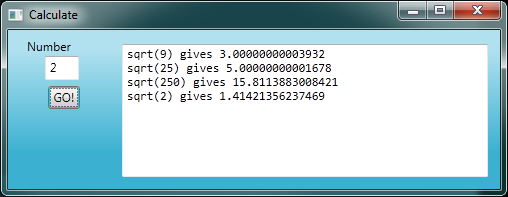• The `while` loop at line 4 has a strange stopping condition — always true! This means it never stops, unless we use a `break` or a `return` to leave the loop. This is what we’ve done at line 9. Returning from the method, even if we’re in the middle of some iteration, happens immediately. The loop does no more iterations.
• The `while (true) { ... }` form of the loop is widely used. This is a language idiom — a convention that most programmers will recognize and understand immediately.
• For handling the GUI, we used a text box to allow the user to enter a number. The code at line 17 shows how we extract the text from the text box, and convert it into a double number before calling our new method.

See if you can improve the approximations by changing the stopping condition. Also, step through the algorithm (perhaps by hand, using your calculator) to see how many iterations are needed before it achieves this level of accuracy for `sqrt(25)`.

Here we’ve made some changes to the loop termination condition: we only stop when the two values are less than 0.00000001 from each other. These results are more accurate now: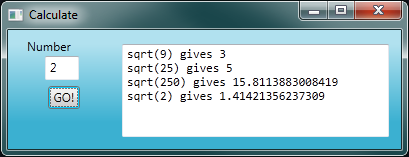## 11.21. Algorithms¶

Newton’s way of getting the answer is an example of an algorithm: it is a mechanical process for solving a category of problems (in this case, computing square roots).

Some kinds of knowledge are not algorithmic. For example, learning dates from history or your multiplication tables involves memorization of specific solutions.

But the techniques you learned for addition with carrying, subtraction with borrowing, and long division are all algorithms. Or if you are an avid Sudoku puzzle solver, you might have some specific set of steps that you always follow.

One of the characteristics of algorithms is that they do not require any intelligence to carry out. They are mechanical processes in which each step follows from the last according to a simple set of rules. And they’re designed to solve a general class or category of problems, not just a single problem.

Understanding that hard problems can be solved by step-by-step algorithmic processes (and having technology to execute these algorithms for us) is one of the major breakthroughs that has had enormous benefits. So while the execution of the algorithm may be boring and may require no intelligence, algorithmic or computational thinking — i.e. using algorithms and automation as the basis for approaching problems — is rapidly transforming our society. Some claim that this shift towards algorithmic thinking and processes is going to have even more impact on our society than the invention of the printing press. And the process of designing algorithms is interesting, intellectually challenging, and a central part of what we call programming.

Some of the things that people do naturally, without difficulty or conscious thought, are the hardest to express algorithmically. Understanding natural language is a good example. We all do it, but so far no one has been able to explain how we do it, at least not in the form of a step-by-step mechanical algorithm.

## 11.22. Glossary¶

algorithm
A step-by-step process for solving a category of problems.
block of statements
A block is a group of statements, usually enclosed in braces.
body
The statements inside a loop. Usually a loop body is a block.
breakpoint
A place in your program code where program execution will pause (or break), allowing you to inspect the state of the program’s variables, or single-step through individual statements, executing them one at a time.
bump
Programmer slang that means increment.
compound statement
see block of statements.
continue statement
A statement that causes the remainder of the current iteration of a loop to be skipped. The flow of execution goes back to the top of the loop, evaluates the condition, and if this is true the next iteration of the loop will begin.
counter
A variable used to count something, usually initialized to zero and incremented in the body of a loop.
decrement
Decrease by 1.
definite iteration
A loop where we have an upper bound on the number of times the body will be executed. Definite iteration is usually best coded using a `for` loop.
encapsulate
To divide a large complex program into components (like methods) and isolate the components from each other (by using local variables, for example).
generalize
To replace something unnecessarily specific (like a constant value) with something appropriately general (like a variable or parameter). Generalization makes code more versatile, more likely to be reused, and sometimes even easier to write.
increment
Used both as a noun and as a verb. The verb means to add to something. The noun is used like this: “Your study fees increment this year will be 8%”.
indefinite iteration
A loop where we just need to keep going until some condition is met. A `while` statement is good to use in this situation.
infinite loop
A loop in which the terminating condition is never satisfied.
initializer
An expression that gives an initial value to a variable where the variable is first defined.
initialization (of a variable)
To initialize a variable is to give it an initial value. In C#, if you don’t do this yourself, your variables will be given default initial values.
iteration
Repeated execution of a set of programming statements.
loop
The construct that allows allows us to repeatedly execute a statement or a group of statements (a block or a compound statement) until a terminating condition is satisfied.
loop variable
A variable used as part of the terminating condition of a loop.
nested loop
A loop inside the body of another loop.
pre-test loop
A loop that tests before deciding whether the execute its body. `for` and `while` are both pre-test loops.
single-step
A mode of execution where you are able to execute your program one step at a time, and inspect the consequences of that step. Useful for debugging and building your internal mental model of what is going on.
trace
To follow the flow of execution of a program by hand, recording the change of state of the variables and any output produced.

## 11.23. Exercises¶

This chapter showed us how to sum an array of items, and how to count items. The counting example also had an `if` statement that let us only count some selected items. In the previous chapter we also showed a method `find_first_2_letter_word` that allowed us an “early exit” from inside a loop by using `return` when some condition occurred. We now also have `break` to exit a loop (but not the enclosing method, and `continue` to abandon the current iteration of the loop without ending the loop.

Composition of array traversal, summing, counting, testing conditions and early exit is a rich collection of building blocks that can be combined in powerful ways to create many methods that are all slightly different.

The first six questions are typical methods you should be able to write using only these building blocks.

1. Write a method to count how many odd numbers are in an array.

2. Sum up all the even numbers in an array.

3. Sum up all the negative numbers in an array.

4. Count how many words in an array have length 5. (Use help to find out how to determine the length of a string.)

5. Sum all the elements in an array up to but not including the first even number. (Write your unit tests. What about the case when there is no even number?)

6. Count how many words occur in an array up to and including the first occurrence of the word “sam”. (Write your unit tests for this case too. Do something sensible if “sam” does not occur.)

7. Add a print statement to Newton’s `sqrt` method to show `better` each time it is calculated. Call your modified method with 25 as an argument and record the results.

8. Trace the execution of the last version of `generateTable` and make yourself more comfortable with single stepping, and debugging.

9. Write a method `print_triangular_numbers(int n)` that prints out the first n+1 triangular numbers. A call to `print_triangular_numbers(5)` would produce the following output:

```0        0
1        1
2        3
3        6
4       10
5       15
```

(Hint: use a web search to find out what a triangular number is.)

1. What happens if we call our Collatz sequence generator with a negative integer?
2. What happens if we call it with zero?
3. Change the method so that it outputs an error message in either of these cases, and doesn’t get into an infinite loop.
10. Write a method, `isPrime`, which takes a single integer argument and returns `true` when the argument is a prime number and `false` otherwise. Add tests for cases like this:

```// 1 is not regarded as prime. It does not have two distinct factors. Ask Google.
Tester.TestEq(isPrime(1), false);
Tester.TestEq(isPrime(2), true);
Tester.TestEq(isPrime(11), true);
Tester.TestEq(isPrime(25), false);
Tester.TestEq(isPrime(35), false);
Tester.TestEq(isPrime(101), true);
Tester.TestEq(isPrime(19951123), true);
Tester.TestEq(isPrime(19961107), true);
Tester.TestEq(isPrime(19951121), false);
Tester.TestEq(isPrime(19961007), false);
```

The last cases could represent your birth date. Were you born on a prime day? In a class of 100 students, how many do you think would have prime birth dates?

11. A drunk pirate makes a turn, and then takes some steps forward, and repeats this. A social science student records pairs of data: the angle of each turn, and the number of steps taken after the turn. Her experimental data is turned into C# as

```int[] turns = {160, -43, 270, -43};
int[] steps = {20, 10, 8, 12 };
```

Use a turtle to draw the path taken by our drunk friend.

12. Many interesting shapes can be drawn by the turtle by giving a pair of arrays of like we did above, where the first array holds the angles to turn, and the second array holds the distances to move forward. Set up a void method that takes a turtle and two arrays, and make the turtle draw the shape defined by the arrays. Then call it so that the turtle draws a house with a cross through the centre, as show here. This should be done without going over any of the lines / edges more than once, and without lifting your pen.13. Not all shapes like the one above can be drawn without lifting your pen, or going over an edge more than once. Which of these can be drawn?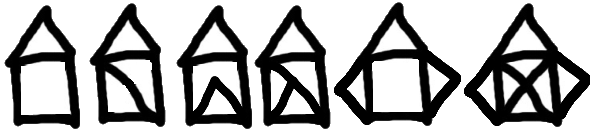Now read Wikipedia’s article(http://en.wikipedia.org/wiki/Eulerian_path) about Eulerian paths. Learn how to tell immediately by inspection whether it is possible to find a solution or not. If the path is possible, you’ll also know where to put your pen to start drawing, and where you should end up!

14. What will `num_digits(0)` return? Modify it to return `1` for this case. Does a call to `num_digits(-12345)` work? Trace through the execution and see what happens if you start with a negative number. Modify `num_digits` so that it works correctly with any integer value. Add these tests:

```Tester.TestEq(num_digits(0), 1);
Tester.TestEq(num_digits(-12345), 5);
```
15. Without making use of strings, write a method `num_even_digits(n)` that counts the number of even digits in `n`. Here are some tests that should pass:

```Tester.TestEq(num_even_digits(123456), 3);
Tester.TestEq(num_even_digits(2468), 4);
Tester.TestEq(num_even_digits(1357), 0);
// Normally we never put leading zeros on numbers, but our number
// system has a special case that probably needs special case handling in the code.
Tester.TestEq(num_even_digits(0), 1);
Tester.TestEq(num_even_digits(0002468), 4)
Tester.TestEq(num_even_digits(-12345), 2);
Tester.TestEq(num_even_digits(-2468), 4);
```
16. Write a method `sum_of_squares(xs)` that computes the sum of the squares of the numbers in the array `xs`. For example, `sum_of_squares(new double[] {2, 3, 4})` should return 4+9+16 which is 29:

```Tester.TestEq(sum_of_squares(new double[] {2, 3, 4}), 29);
Tester.TestEq(sum_of_squares(new double[] {}), 0);
Tester.TestEq(sum_of_squares(new double[] {2, -3, 4}), 29);
```